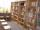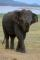# Bureau of Labor

Bureau of Labor is a state institution that provides mike and the rest for their so-called clients. The mission of the Bureau of Labor is spend taxpayer money to provide relaxation and benefits to those who do not want to work. Popularly speaking sense the work of the Bureau of Labor is to create the largest number of unemployed. This helps him massively Labour Code, trade unions, minimum wage and other regulations that were not here.

Back to mathematics. Probability that Bureau of Labor "finds" unemployed job is 1.7%. Probability that a job seeker finds job as active person is 93%.

Calculate how many times the probability of finding a job more his own efforts than through Bureau of Labor.

Result

x =  54.7

#### Solution:Leave us a comment of example and its solution (i.e. if it is still somewhat unclear...):

Showing 0 comments:Be the first to comment!#### To solve this example are needed these knowledge from mathematics:

Need help calculate sum, simplify or multiply fractions? Try our fraction calculator. Our percentage calculator will help you quickly calculate various typical tasks with percentages. Would you like to compute count of combinations?

## Next similar examples:

1. WithdrawalIf I withdrew 2/5 of my total savings and spent 7/10 of that amount. What fraction do I have in left in my savings?
2. TeacherTeacher Rem bought 360 pieces of cupcakes for the outreach program of their school. 5/9 of the cupcakes were chocolate flavor and 1/4 wete pandan flavor and the rest were vanilla flavor. How much more chocolate flavor cupcakes than vanilla flavor?
3. School libraryThe school library contains 6300 books. With this constitutes 7% of professional books for teachers 18% interest books and encyclopedias for pupils and the rest of the fairy tales. How much are which books?
4. Fraction to decimalWrite the fraction 3/22 as a decimal.
5. Pizza 5You have 2/4 of a pizza and you want to share it equally between 2 people how much pizza does each person get?
6. ClassIn 7.C clss are 10 girls and 20 boys. Yesterday was missing 20% of girls and 50% boys. What percentage of students missing?
7. ZdeněkZdeněk picked up 15 l of water from a 100-liter full-water barrel. Write a fraction of what part of Zdeněk's water he picked.
8. Product of two fractionsProduct of two fractions is 9 3/5 . If one of the fraction is 9 3/7. Find the other fraction.
9. In fractionsAn ant climbs 2/5 of the pole on the first hour and climbs 1/4 of the pole on the next hour. What part of the pole does the ant climb in two hours?
10. Ratio 11Simplify this ratio 10 : 1/4
11. Write 2Write 791 thousandths as fraction in expanded form.
12. Lengths of the poolMiguel swam 6 lengths of the pool. Mat swam 3 times as far as Miguel. Lionel swam 1/3 as far as Miguel. How many lengths did mat swim?
13. Seeds 2How many seeds germinated from 1000 pcs, when 23% no emergence?
14. PointsGryffindor won 437 points. How many points obtained by each of the faculties if they were split at a ratio of 5: 7: 3: 4?
15. AndreAndre, Thomas, and Ivan split 88 postage stamps in a 2:5:4 ratio. How much did Thomas get?
16. Fractions 4How many 2/3s are in 6?
17. 100 %How much is 100%, if 17 % is 1169?